## Abstract

In the last 15 years, Double Fed Induction Generator (DFIG) had been widely used as a wind turbine generator, due its various advantages especially low generation cost so it becomes the most important and promising sources of renewable energy. This work focuses on studying of using DFIG as a wind turbine connected to a grid subjected to various types of fault. Crowbar is a kind of protection used for wind turbine generator protection. ANFIS controller is used for protection of DFIG during faults. The fault current under symmetric and asymmetric fault is presented as well as a way to control the increase in rotor current which leads to voltage increase in DC link between wind generator and the grid. ANFIS is used for solving such problem as it is one of the most commonly AI used techniques. Also the current response of DFIG during fault is improved by adapting the parameters of PI controllers of the voltage regulator using fuzzy logics. ANFIS also in this paper is used for detecting and clearing the short circuit on the DC capacitor link during the operation. A simulation study is illustrated using MATLAB/Simulink depending on currents and voltages measurement only for online detection of the faults. The proposed technique shows promising results using the simulation model.

## Keywords

Double Fed Induction Generator; Crowbar protection; ANFIS; Fuzzy logic; Fault current and voltages

## 1. Introduction

Wind power generation industry has become widely used in the last few years and takes more attention of manufactures. There are many reasons for adding more wind energy to the electric networks. For instance, wind generation is supported by not only being clean and renewable but also having minimal running cost requirements .

Variable speed wind turbine topologies include many different generator/converter configurations, based on cost, efficiency, annual energy capturing, and control complexity of the overall system .

Due to the fast enhancement and development in manufacture of power electronic converter technology as well as the development of induction machines specially Double Fed Induction Generators and its advantages of small capacity of converters, high energy and flexible power control , DFIG has been widely used for large-scale wind power generation systems due to its various advantages, such as variable speed operation, controllable power factor and improved system efficiency . The amount of energy extracted from the wind depends not only on the incident wind speed, but also on the control system applied on the wind energy conversion system. The DFIG is equipped with a back-to-back power electronic converter, which can adjust the generator speed with the variety of wind speed. The converter is connected to the rotor windings, which acts as AC excitation system. Wind turbines began to contribute and increase steadily in electric power generation production in electric networks.

The power electronic converter between the stator and the grid as in variable speed wind turbine can determine the short circuit fault current easily, in DFIG in either constant speed or variable speed wind turbines and the converter is connected between the rotor winding and the grid and exposed to high current during fault occurrence .

The contribution of DFIG wind turbine in the fault current must be taken into account as the wind farm must stay connected to the grid during system disturbances in order to support the network voltage and frequency. Some precaution must be taken into account to ensure the safe operation of the rotor side inverter of the DFIG, as the rotor current will increase during grid failures. Therefore, DFIG requires a protection system named active crowbar disconnects the converter in order to protect it turning the generator into a squirrel cage induction machine  and . Till now the crowbar protection still takes the attention of many researchers trying to study its effects for both the grid and the wind turbine, and also many papers have studied only the effect of fault on the DFIG ,  and , as well as many types of crowbar protections had been introduced which divide the crowbar protection system into three types, conventional crowbar, series crowbar and a new protection method, named the outer crowbar, as this type was presented in  similar to the series crowbar but the main difference between the series crowbar and the outer series crowbar is the outer crowbar connects in series with the DFIG instead of stator windings only. According to the position of crowbar resistance with respect to either stator winding or rotor winding side , the amount and the direction of power flowing through the rotor circuit depend on the operating point of the induction machine.

Two control schemes are developed for rotor- and grid-side converters. Shunt capacitor is employed as a dc link between the two converters. Ref.  shows the behavior of DFIG in case of capacitor failure. However, none of the previous work introduces a solution for this problem. Ref.  presents different fault conditions such as line to ground faults, line to line faults, double line to ground faults and three phase symmetric faults using genetic algorithm based fuzzy controller is incorporated into the Doubly fed Induction Generator (DFIG) Wind Energy Conversion System. While the behaviors of DFIG wind turbine under micro-interruption fault are studied in , the micro-interruption is a disconnection of the electric grid for a short moment where a control strategy of the Unified Power Flow Control (UPFC) using PI controller is presented. Different kinds of research are applied on the crowbar of the DFIG as indicated in , , , ,  and  but none of them used ANFIS for DFIG protection. ANFIS controller was implemented for MPPT of DFIG .

This paper presents a new technique of crowbar implementation using ANFIS controller. The response of DFIG during different types of faults is improved by adapting the parameters of PI controllers of the voltage regulator using fuzzy logics. ANFIS is also used for detecting and clearing the short circuit on the DC capacitor link during the operation. The dynamics of the system and control actions are simulated with detailed model using MATLAB/SIMULINK. This paper is divided into six sections. First section is an introduction; second section is representing the model used in Simulink. The equations of DFIG are studied in Section3 3, Section 4 shows the fuzzy logic algorithm, Section 5 is the simulation results and finally Section 6 represents the conclusion.

## 2. System under study

The studied system consists of DFIG (575 V, 10 MW) connected to a grid (2500 MVA) through a transmission line system of 30 km, 25 kV, step-up and down transformers as shown in Fig. 1. The representation of such networks containing DFIG working as a wind turbine is done through representing the mathematical equations that describe the dynamic methodology of wind energy conversion to electrical energy. Wind energy conversion system consists of two main parts: wind turbine with the pitch regulator and the induction generator each can be described as follows: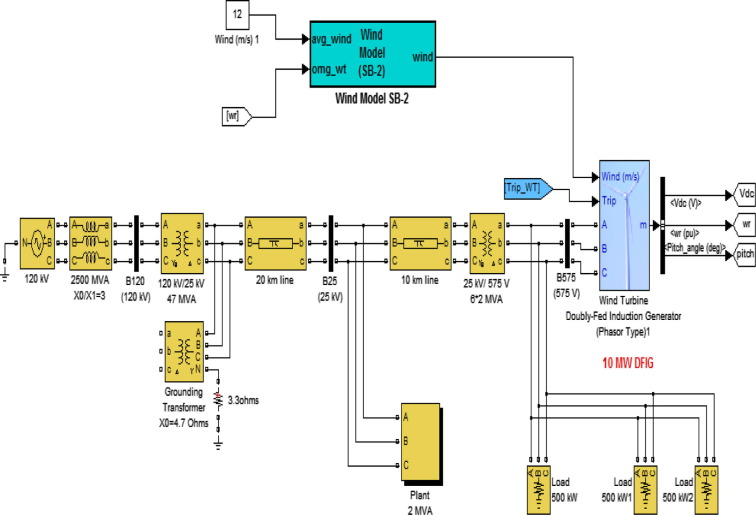Figure 1. Wind turbine connected to grid.

### 2.1. Wind turbine with regulator pitch model

The modeling of this module is depending on the representation of the aerodynamic power captured from the wind and how much wind energy is converted to mechanical energy, so the mathematical equations representing it will relate to the output power directly to the air flow as follows :

 $P_{w}={\frac {1}{2}}\rho C_{p}(\lambda {\mbox{,}}\beta )A_{R}v_{w}^{3}$
(1)
 $C_{p}(\lambda {\mbox{,}}\beta )=0.22(116/{\lambda }_{i}-$$0.4\beta -5.0)e^{\frac {-12.5}{{\lambda }_{i}}}$
(2)
 ${\lambda }_{i}={\left({\frac {1}{\lambda +0.08\beta }}-{\frac {0.035}{{\beta }^{3}+1}}\right)}^{-1}$
(3)

where Pw is the aerodynamic power captured from wind; ρ is the density of air kg/m3; AR is the area that the wind power can be obtained; Cp is the power coefficient; vw is the wind speed; λ is the tip speed ratio (TSR); λi is the middle variable; and β is the pitch angle. The main construction of DFIG model is indicated in Fig. 2.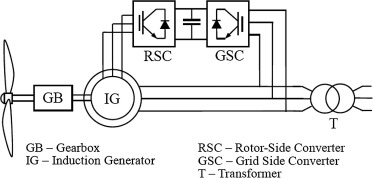Figure 2. Construction of DFIG.

## 3. Induction generator model

For the mechanical model, it was concentrated only on the parts of the dynamic structure of the wind turbine that contributes to the interaction with the grid . Therefore, only the drive train is considered, while the other parts of the wind turbine structure, e.g. tower and flap bending modes, are neglected. When modeling the drive train, it is a common practice to neglect the dynamic, of the mechanical parts, as their responses are considerably slow in comparison with the fast electrical ones, especially for machines with great inertia. The rotational system may therefore be modeled by a single equation of motion:

 $J_{WG}{\frac {{dw}_{r}}{dt}}=T_{W}-T_{G}-{Dw}_{r}$
(4)

where ${\textstyle J_{WG}}$ is the wind turbine mechanical inertia plus generator mechanical inertia [kg m2], Tw is mechanical torque. TG is generator electromagnetic torque [N m], and Distraction coefficient [N m/rad].

Also the equivalent circuit of DFIG model is shown in Fig. 3.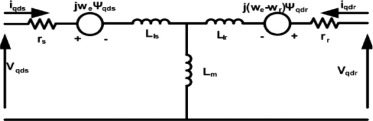Figure 3. Equivalent circuit of DFIG.

This model can be described by the following space vector equations in synchronous coordinates as follows:

 $u_{sd}=R_{s}i_{sd}+{\frac {d}{dt}}{\Psi }_{sd}-{\omega }_{1}{\Psi }_{sq}$
(5)
 $u_{sq}=R_{s}i_{sq}+{\frac {d}{dt}}{\psi }_{sq}+{\omega }_{1}{\psi }_{sd}$
(6)
 $u_{rd}=R_{r}i_{rd}+{\frac {d}{dt}}{\psi }_{rd}-{\omega }_{1}{\psi }_{rq}$
(7)
 $u_{rq}=R_{r}i_{rq}+{\frac {d}{dt}}{\psi }_{rq}+{\omega }_{1}{\psi }_{rd}$
(8)
 ${\Psi }_{sd}=L_{s}i_{sd}+L_{m}i_{rd}$
(9)
 ${\Psi }_{sq}=L_{s}i_{sq}+L_{m}i_{rq}$
(10)
 ${\Psi }_{rd}=L_{m}i_{sd}+L_{r}i_{rd}$
(11)
 ${\Psi }_{sq}=L_{m}i_{sq}+L_{r}i_{rq}$
(12)

where Rs, Rr are stator, rotor resistance, LS, Lr are stator, rotor leakage inductance, Lm is magnetizing inductance, μs, μr are stator, rotor voltage, and is, Ir are stator rotor Current . W1 is synchronous angular speed. When the d reference frame synchronous coordinate was oriented along with the stator flux of the DFIG,

The active and reactive power equations at the stator and rotor windings are written as follows:

 $Ps=Vds\quad ids+Vqs\quad iqs$
(13)
 $Qs=Vqs\quad ids-Vds\quad iqs$
(14)
 $Pr=Vqr\quad idr+Vqr\quad iqr$
(15)
 $Qr=Vdr\quad idr-Vdr\quad iqr$
(16)

The electromagnetic torque is expressed as follows:

 $T_{em}={\frac {3}{2}}{_{\ast }}{\frac {p}{2}}({\varphi }_{ds}{_{\ast }}i_{qs}-$${\varphi }_{qs}i_{ds})$
(17)

## 4. Fuzzy logic control algorithm

The use of fuzzy logic control (FLC) has become popular over the last decade because it can deal with imprecise inputs, does not need an accurate mathematical model and can handle nonlinearity. Microcontrollers have also helped in the popularization of FLC. Neural networks are the systems that get inspiration from biological neuron systems and mathematical theories for learning. They are characterized by their learning ability with a parallel-distributed structure .

An Adaptive Neuro-Fuzzy Inference System (ANFIS) is a cross between an Artificial Neural Network (ANN) and a fuzzy inference system (FIS) as the main purpose of using the Neuro-Fuzzy approach is to automatically realize the fuzzy system by using the neural network methods. An adaptive network is a multi-layer feed-forward network in which each node (neuron) performs a particular function on incoming signals. The form of the node functions may vary from node to node. In an adaptive network, there are two types of nodes, adaptive and fixed.

In this paper a novel contribution through using ANFIS is achieved to reach two main goals, first to detect and protect the rotor winding of wind turbine generator when subjected to fault. Second to implement a standby DC capacitor in operation in case of the original capacitor is damaged due to voltage rise on it during fault subjection. Also fuzzy logic controller is used for PI tuning adaption of controller parameter to achieve better response.

The implementation of crowbar using ANFIS technique for the wind turbine during grid faults is presented. The fault locations on the grid are indicated in Fig. 4.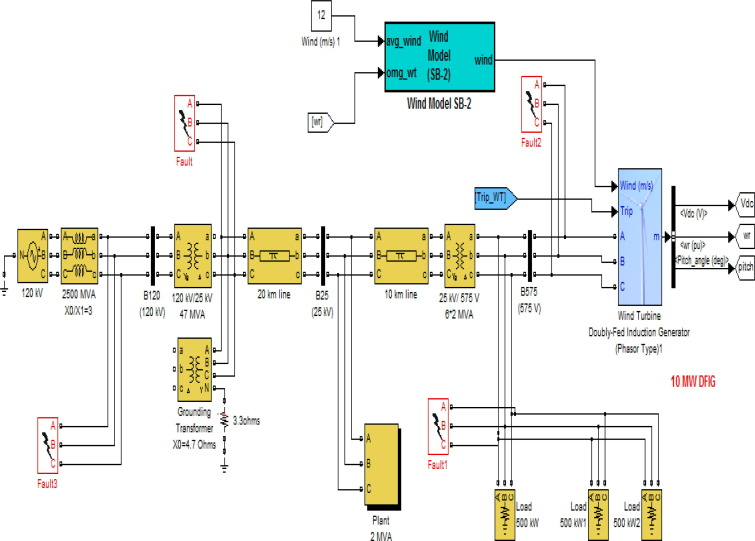Figure 4. Locations of faults on the grid.

### 4.1. ANFIS design for crowbar

The main purpose of using ANFIS controller in this paper was for implementation of crowbar resistance in the rotor side of wind turbine during grid fault. ANFIS technique is shown in Fig. 5, Fig. 6 shows the standby capacitor implementation during operation using ANFIS technique. The ANFIS controller structure is shown in Fig. 7, and the outputs of the ANFIS controller have three values: Zero to indicate no fault occurrence, one for fault occurrence, and two for capacitor short circuit.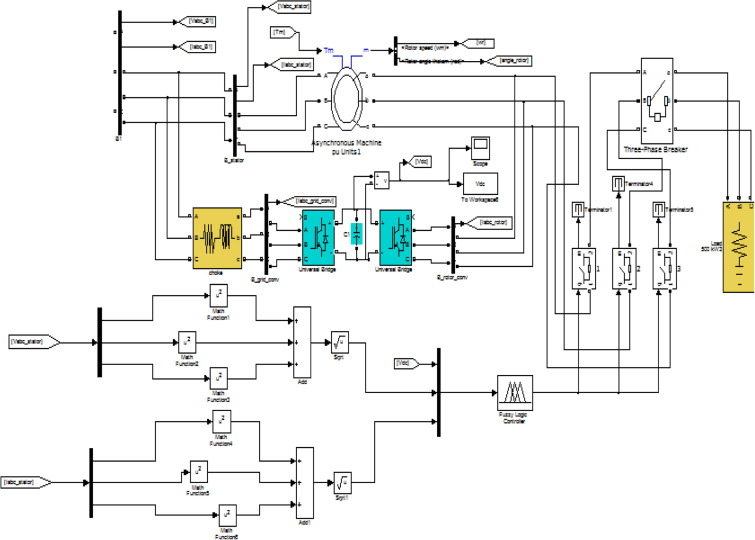Figure 5. ANFIS technique for crowbar protection.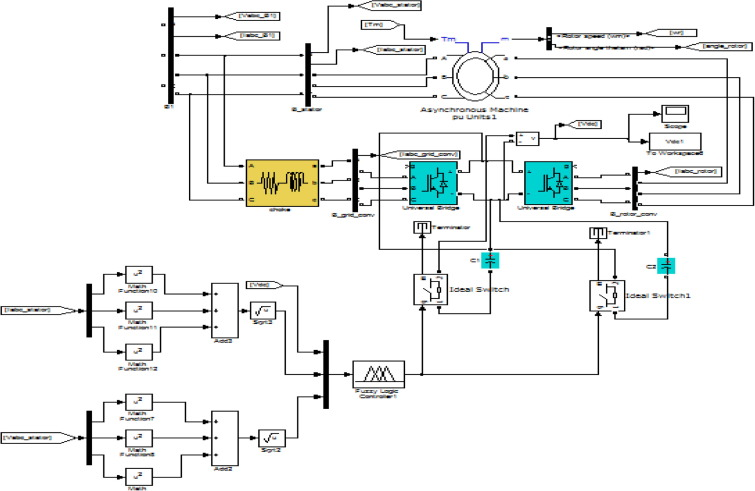Figure 6. Implementation of ANFIS for capacitor short circuit.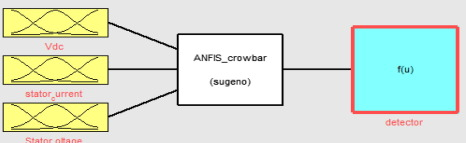Figure 7. ANFIS inputs/outputs for crowbar protection.

The inputs of ANFIS controller are as follows:

• VDC
• Stator current Is = sqrt(Isa2 + Isb2 + Isc2)
• Stator voltage Vs = sqrt(Vsa2 + Vsb2 + Vsc2)

The fuzzy logic observer parameters are turned using neural network method which is well known in MATLAB program as ANFIS structure. The parameters are selected such that, optimization method is hybrid, the membership function is gbellmf, the membership function output is linear, error tolerance was chosen to be 0.01, the number of epochs is 1000, grid partitions, the inputs of the grid partitions are the number MFS are 3, MF type is gbellmf, the outputs is MF type defined to be constant.

### 4.2. PI adaptation using fuzzy logic controller

This paper proposed two inputs-two outputs self-tuning of a PI controller. The controller design used the error and change of error as inputs to the self-tuning, and the gains (${\textstyle K_{P1}{\mbox{,}}K_{I1}}$) as outputs. The FLC is adding to the conventional PID controller to adjust the parameters of the PI controller online according to the change of the signals error and change of the error. The proposed controller also contains a scaling gains inputs (ek, Δek) as shown in Fig. 8, to satisfy the operational ranges (the universe of discourse) making them more general.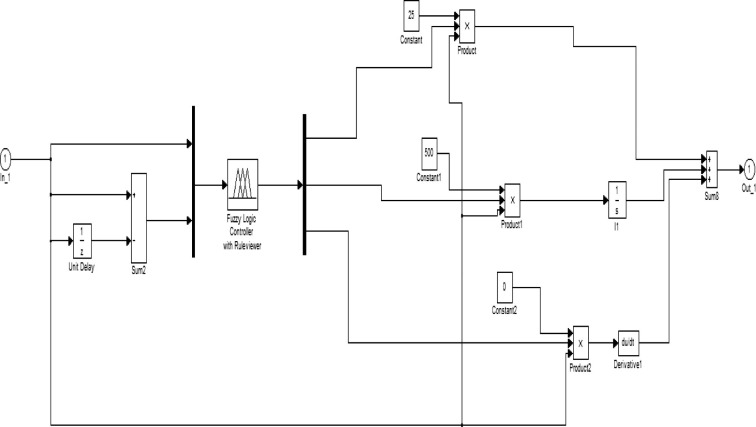Figure 8. Adaptation of PI controller using fuzzy logic.

Now the control action of the PID controller after self-tuning can be described as follows:

 $U_{PID}=K_{P2}{_{\ast }}e(t)+K_{I2}\int edt$
(18)

where ${\textstyle K_{P2}{\mbox{,}}K_{I2}}$, are the new gains of PI controller and are equal to the following:

 $K_{P2}=K_{P1}{_{\ast }}K_{P}{\mbox{,}}\quad K_{I2}=K_{I1}{_{\ast }}K_{I}$
(19)

where ${\textstyle K_{P1}}$ and ${\textstyle K_{I1}}$, are the gains outputs of fuzzy control, that are varying online with the output of the system under control. And ${\textstyle K_{P}}$ and ${\textstyle K_{I}}$, are the initial values of the conventional PID.

For the system under study the universe of discourse for both e(t) and Δe(t) may be normalized from [−1, 1], and the linguistic labels are {Negative Big, Negative, medium, Negative small, Zero, Positive small, Positive medium, Positive Big }, and are referred to in the rules bases as {NB, NM, NS, ZE, PS, PM, PB}, and the linguistic labels of the outputs are {Zero, Medium small, Small, Medium, Big, Medium big, very big} and referred to in the rules bases as {Z, MS, S, M, B, MB, VB} as shown in Figure 9 and Figure 10.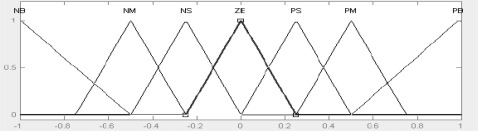Figure 9. Membership functions of inputs (e, Δe).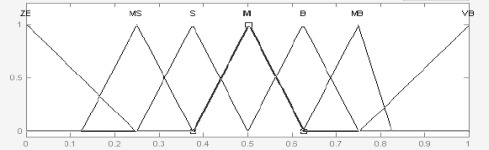Figure 10. Membership functions of outputs (KP1, KI1).

## 5. Simulations results

The simulations are done using the model shown in Fig. 4 through subjecting the system to different faults in different places. The first fault is a triple line to ground fault at the wind turbine side occurring during the first duration (0.2–0.4 s), second fault is double line to ground fault at the loads during the second duration (0.6–0.8 s), and third fault located at the plant (2 MVA) is triple line to ground fault occurring during the third duration (1–1.2 s). Finally a single line to ground fault occurs before transformer (47 MVA) during the fourth duration (1.4–1.9 s). The DC capacitor link is also shorted during the fifth duration (0.12–0.17 s). Fig. 11 presents the ANFIS output during grid faults from the 1st to 5th duration indicating by 1 or in other word a fault is detected and then cleared and returned back to its normal operation (0), and also the DC capacitor short circuit at the 5th duration at which the ANFIS output gives value two and the fault is cleared and returns back to its normal value.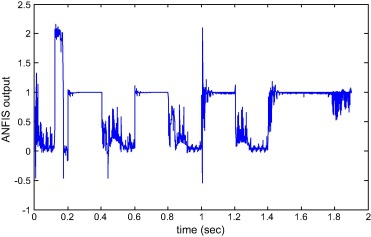Figure 11. ANFIS output during grid faults and DC capacitor shorted.

Fig. 12 shows the change of the DC capacitor voltage during capacitor short circuit fault beginning with nominal value 1200 V and decreasing to approximate zero value and the returning back to its normal value after clearing the fault.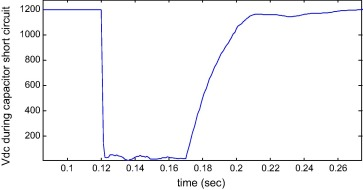Figure 12. Vdc during capacitor short circuit.

Fig. 13 shows the rise in Vdc without using the crowbar protection and how this value is reduced when using ANFIS crowbar, and also Fig. 14 shows the high oscillation and over shoots appear in the stator current in case of not using ANFIS controller and how this control algorithm succeeds in damping the output signal to reach its nominal value in a few seconds.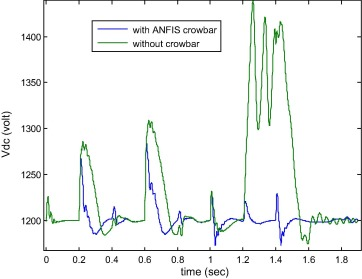Figure 13. Vdc with and without crowbar during faults.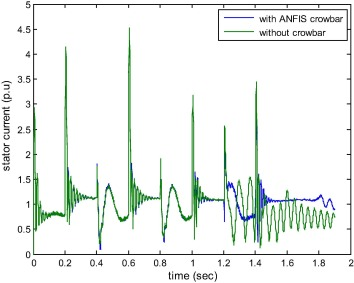Figure 14. Stator current with and without crowbar during faults.

Figure 15 and Figure 16 represent the enhancement achieved for Vdc and the stator current in reducing the overshoots and oscillation damping due to implementation of PI adaptation using fuzzy logic controller compared to the conventional PI.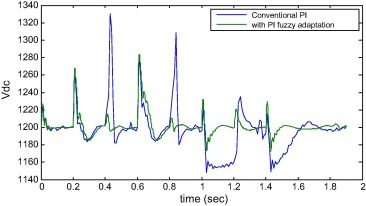Figure 15. Vdc with and without PI adaptation during faults.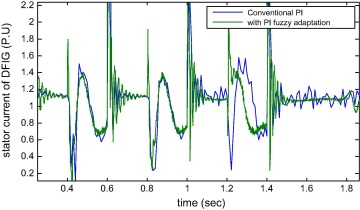Figure 16. Stator current with and without PI adaptation during faults.

## 6. Conclusion

The short circuit current fault analysis in DFIG wind turbine connected to a grid still takes the attention of many researchers. This paper succeeds in studying its effect when subjected to various types of faults for both the grid and the wind turbine also in designing such controller based on AI optimization technique which shows greet advance in controller output as shown in simulation results.

This paper also presents a new technique of crowbar implementation using ANFIS controller as well as the response of DFIG during different types of faults is improved by adapting the parameters of PI controllers of the voltage regulator using fuzzy logic controller.

Finally the ANFIS optimization technique is succeeded in detecting and clearing the failure of the DC capacitor link during operation.

### Document informationPublished on 12/04/17

Licence: Other

### Document Score0

Views 272
Recommendations 0